一、线性表
1.数组实现
2.链表

1.树
2.二叉树基本概念
3.二叉查找树
4.平衡二叉树
5.红黑树



### 一、线性表

##### 数组实现

• 代码1 创建一个更大的数组来替换当前数组
int[] oldArray = new int;

int[] newArray = new int;

for (int i = 0; i < oldArray.length; i++) {
newArray[i] = oldArray[i];
}

// 也可以使用System.arraycopy方法来实现数组间的复制
// System.arraycopy(oldArray, 0, newArray, 0, oldArray.length);

oldArray = newArray;

• 代码2 在数组位置index上添加元素e
//oldArray 表示当前存储元素的数组
//size 表示当前元素个数
public void add(int index, int e) {

if (index > size || index < 0) {
System.out.println("位置不合法...");
}

//如果数组已经满了 就扩容
if (size >= oldArray.length) {
// 扩容函数可参考代码1
}

for (int i = size - 1; i >= index; i--) {
oldArray[i + 1] = oldArray[i];
}

//将数组elementData从位置index的所有元素往后移一位
// System.arraycopy(oldArray, index, oldArray, index + 1,size - index);

oldArray[index] = e;

size++;
}


##### 链表• 代码3 链表的节点
class Node<E> {

E item;
Node<E> next;

//构造函数
Node(E element) {
this.item = element;
this.next = null;
}
}

• 代码4 定义好节点后，使用前一般是对头节点和尾节点进行初始化
//头节点和尾节点都为空 链表为空
Node<E> tail = null;

• 代码5 空链表创建一个新节点
//创建一个新的节点 并让head指向此节点

//让尾节点也指向此节点

• 代码6 链表追加一个节点
//创建新节点 同时和最后一个节点连接起来
tail.next = new Node("node1data2");

//尾节点指向新的节点
tail = tail.next;

• 代码7 顺序遍历链表
Node<String> current = head;
while (current != null) {
System.out.println(current.item);
current = current.next;
}

• 代码8 倒序遍历链表
static void printListRev(Node<String> head) {
//倒序遍历链表主要用了递归的思想
}
}

• 代码 单链表反转
//单链表反转 主要是逐一改变两个节点间的链接关系来完成

return null;
}

Node<String> nodeResult = null;

Node<String> nodePre = null;

while (current != null) {

Node<String> nodeNext = current.next;

if (nodeNext == null) {
nodeResult = current;
}

current.next = nodePre;
nodePre = current;
current = nodeNext;
}

return nodeResult;
}


### 二、栈与队列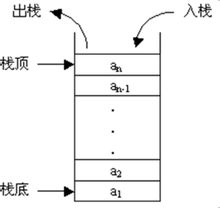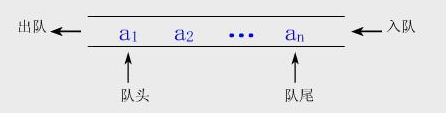• 代码9 简单实现队列类
public class MyQueue<E> {

// 入队
public void enqueue(E e) {
}

// 出队
public E dequeue() {
return list.removeFirst();
}
}


### 三、树与二叉树

** 树 是由n（n>=1）个有限节点组成一个具有层次关系的集合。它具有以下特点：每个节点有零个或多个子节点；没有父节点的节点称为节点；每一个非根节点有且只有一个 父节点 **；除了根节点外，每个子节点可以分为多个不相交的子树。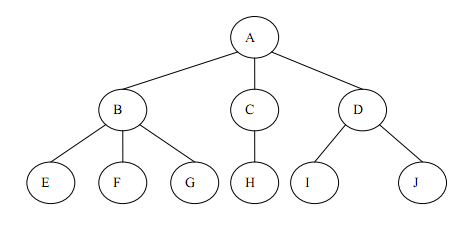• 定义

• 相关性质• 三种遍历方法

(1) 先序遍历 若二叉树为空，则空操作，否则先访问根节点，再先序遍历左子树，最后先序遍历右子树。 (2) 中序遍历 若二叉树为空，则空操作，否则先中序遍历左子树，再访问根节点，最后中序遍历右子树。(3) 后序遍历 若二叉树为空，则空操作，否则先后序遍历左子树访问根节点，再后序遍历右子树，最后访问根节点。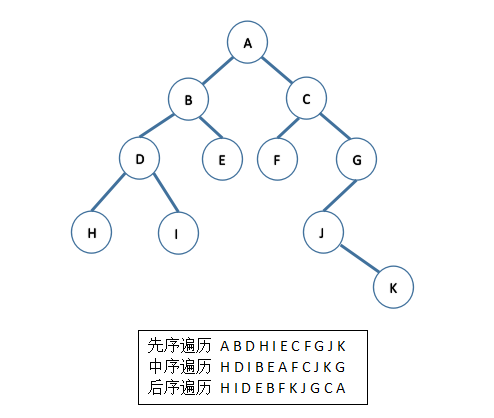• 树和二叉树的区别

(1) 二叉树每个节点最多有2个子节点，树则无限制。 (2) 二叉树中节点的子树分为左子树和右子树，即使某节点只有一棵子树，也要指明该子树是左子树还是右子树，即二叉树是有序的。 (3) 树决不能为空，它至少有一个节点，而一棵二叉树可以是空的。

• 定义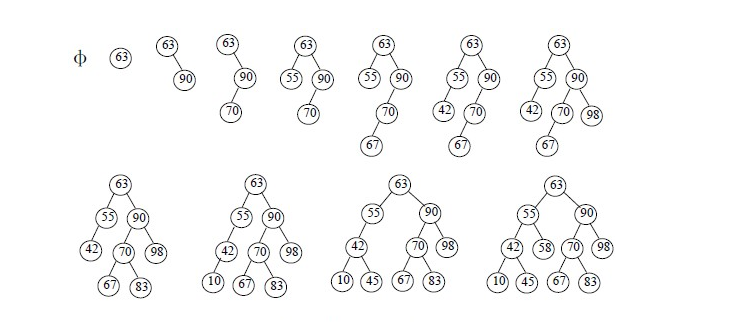• 性能分析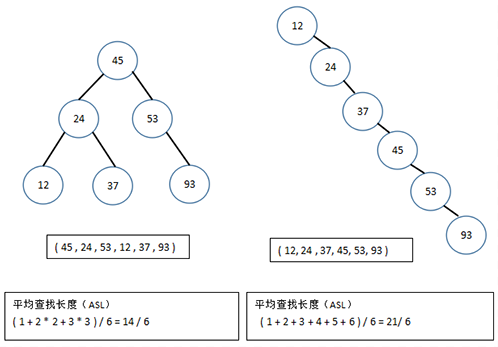• 代码10 二叉树的节点

class TreeNode<E> {

E element;
TreeNode<E> left;
TreeNode<E> right;

public TreeNode(E e) {
element = e;
}
}


• 代码12 先序遍历
protected void preorder(TreeNode<E> root) {

if (root == null)
return;

System.out.println(root.element + " ");

preorder(root.left);

preorder(root.right);
}

• 代码13 中序遍历
protected void inorder(TreeNode<E> root) {

if (root == null)
return;

inorder(root.left);

System.out.println(root.element + " ");

inorder(root.right);
}

• 代码14 后序遍历
protected void postorder(TreeNode<E> root) {

if (root == null)
return;

postorder(root.left);

postorder(root.right);

System.out.println(root.element + " ");
}

• 代码15 二叉查找树的简单实现
/**
* @author JackalTsc
*/
public class MyBinSearchTree<E extends Comparable<E>> {

// 根
private TreeNode<E> root;

// 默认构造函数
public MyBinSearchTree() {
}

// 二叉查找树的搜索
public boolean search(E e) {

TreeNode<E> current = root;

while (current != null) {

if (e.compareTo(current.element) < 0) {
current = current.left;
} else if (e.compareTo(current.element) > 0) {
current = current.right;
} else {
return true;
}
}

return false;
}

// 二叉查找树的插入
public boolean insert(E e) {

// 如果之前是空二叉树 插入的元素就作为根节点
if (root == null) {
root = createNewNode(e);
} else {
// 否则就从根节点开始遍历 直到找到合适的父节点
TreeNode<E> parent = null;
TreeNode<E> current = root;
while (current != null) {
if (e.compareTo(current.element) < 0) {
parent = current;
current = current.left;
} else if (e.compareTo(current.element) > 0) {
parent = current;
current = current.right;
} else {
return false;
}
}
// 插入
if (e.compareTo(parent.element) < 0) {
parent.left = createNewNode(e);
} else {
parent.right = createNewNode(e);
}
}
return true;
}

// 创建新的节点
protected TreeNode<E> createNewNode(E e) {
return new TreeNode(e);
}

}

// 二叉树的节点
class TreeNode<E extends Comparable<E>> {

E element;
TreeNode<E> left;
TreeNode<E> right;

public TreeNode(E e) {
element = e;
}
}



• 二叉查找树中删除节点分析

1. current节点没有左孩子，那么只需要将patent节点和current节点的右孩子相连。
2. current节点有一个左孩子，假设rightMost指向包含current节点的左子树中最大元素的节点，而parentOfRightMost指向rightMost节点的父节点。那么先使用rightMost节点中的元素值替换current节点中的元素值，将parentOfRightMost节点和rightMost节点的左孩子相连，然后删除rightMost节点。
    // 二叉搜索树删除节点
public boolean delete(E e) {

TreeNode<E> parent = null;
TreeNode<E> current = root;

// 找到要删除的节点的位置
while (current != null) {
if (e.compareTo(current.element) < 0) {
parent = current;
current = current.left;
} else if (e.compareTo(current.element) > 0) {
parent = current;
current = current.right;
} else {
break;
}
}

// 没找到要删除的节点
if (current == null) {
return false;
}

// 考虑第一种情况
if (current.left == null) {
if (parent == null) {
root = current.right;
} else {
if (e.compareTo(parent.element) < 0) {
parent.left = current.right;
} else {
parent.right = current.right;
}
}
} else { // 考虑第二种情况
TreeNode<E> parentOfRightMost = current;
TreeNode<E> rightMost = current.left;
// 找到左子树中最大的元素节点
while (rightMost.right != null) {
parentOfRightMost = rightMost;
rightMost = rightMost.right;
}

// 替换
current.element = rightMost.element;

// parentOfRightMost和rightMost左孩子相连
if (parentOfRightMost.right == rightMost) {
parentOfRightMost.right = rightMost.left;
} else {
parentOfRightMost.left = rightMost.left;
}
}

return true;
}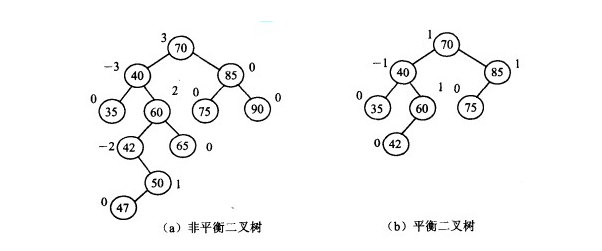AVL树是最先发明的自平衡二叉查找树算法。在AVL中任何节点的两个儿子子树的高度最大差别为1，所以它也被称为高度平衡树，n个结点的AVL树最大深度约1.44log2n。查找、插入和删除在平均和最坏情况下都是O（log n）。增加和删除可能需要通过一次或多次树旋转来重新平衡这个树。

### 四、图

• 简介

• 相关阅读

(1) 《百度百科对图的介绍》
(2) 《数据结构之图（存储结构、遍历）》

### 五、总结## 发送评论编辑评论

|´・ω・)ノ
ヾ(≧∇≦*)ゝ
(☆ω☆)
（╯‵□′）╯︵┴─┴
￣﹃￣
(/ω＼)
∠( ᐛ 」∠)＿
(๑•̀ㅁ•́ฅ)
→_→
୧(๑•̀⌄•́๑)૭
٩(ˊᗜˋ*)و
(ノ°ο°)ノ
(´இ皿இ｀)
⌇●﹏●⌇
(ฅ´ω`ฅ)
(╯°A°)╯︵○○○
φ(￣∇￣o)
ヾ(´･ ･｀｡)ノ"
( ง ᵒ̌皿ᵒ̌)ง⁼³₌₃
(ó﹏ò｡)
Σ(っ °Д °;)っ
( ,,´･ω･)ﾉ"(´っω･｀｡)
╮(╯▽╰)╭
o(*////▽////*)q
＞﹏＜
( ๑´•ω•) "(ㆆᴗㆆ)

Emoji